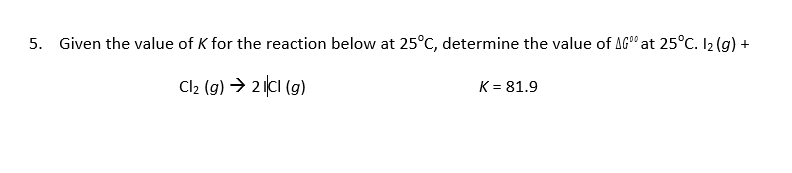# Given the value of K for the reaction below at 25°C, determine the value of AG° at 25°C. I2(g) +5.Cl2 (g)2(cl (g)K-81.9

Question
17 viewshelp_outlineImage TranscriptioncloseGiven the value of K for the reaction below at 25°C, determine the value of AG° at 25°C. I2(g) + 5. Cl2 (g)2(cl (g) K-81.9 fullscreen
check_circle

Step 1

The expression that relates the equilibrium constant of a reaction and change in gibbs free energy of the reaction is:

Step 2

The value of ideal gas constant = R = 8.314 J/K/mol

Temperature = 25 0C = (25+273) K = 298 K

The given value of the ...

### Want to see the full answer?

See Solution

#### Want to see this answer and more?

Solutions are written by subject experts who are available 24/7. Questions are typically answered within 1 hour.*

See Solution
*Response times may vary by subject and question.
Tagged in

### Chemistry